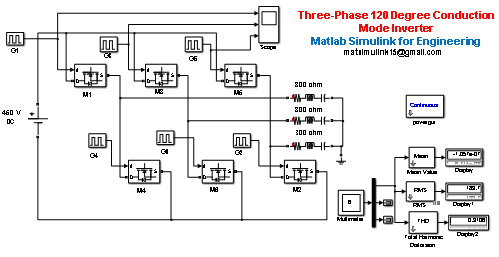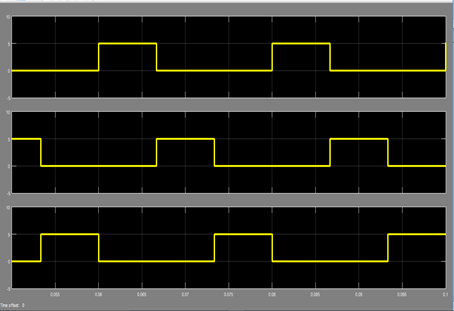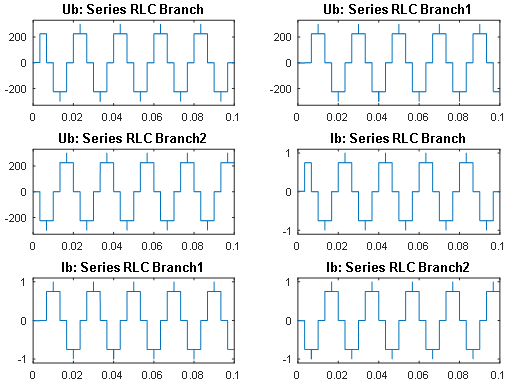3 Phase 120 Degree Mode Inverter using matlab simulink - Matlab Simulink for E & T

## Thursday, July 16, 2020120 Degree Conduction Mode Inverter using matlab simulink

Three-Phase 120 Degree Conduction Mode InverterSimulink Model of Three-Phase 120 Degree Conduction Mode Inverter

# A three phase 120 degree conduction mode bridge type inverter model built up of six MOSFETs, 450 V DC input Supply , Star connected 300 ohm load, six pulse generator,. In this inverter, each MOSFET conducts for 120 degree and hence it is known as three phase VSI 120 Degree Conduction Mode Inverter.

For best laptop for Matlab software-  https://amzn.to/39QeZ9d

Camera- https://amzn.to/39KBi02

Professional tripod- https://amzn.to/39Jg96w

 Angle (Degree) ON Switches OFF Switches 0°-60° M1, M6 M2, M3, M4, M6 60°-120° M1, M2 M2, M3, M4, M5 120°-180° M2, M3 M1, M4, M5, M6 180°-240° M3, M4 M1, M2, M5, M6 240°-300° M4, M5 M1, M2, M3, M6 300°-360° M5, M6 M1, M2, M3, M4

Simulation Time- 0 to 0.1 sec

A period of 0.02 sec is taken so that the output voltage waveform is of frequency 50 Hz (T=1/f, i.e. 1/50=0.02).

The pulse width of 33.34% is taken so that the MOSFET, M1 conducts from 0° to 120° sec .i.e. 0 to 180 degree, i.e. 0 to 0.0067 sec.

Similarly the phase delay

For    G2 it is 0.01/3 sec,

For    G3 it is 0.02/3 sec,

For    G4 it is 0.01 sec.

For   G5 it is 0.04/3, and

For   G6 it is 0.05/3 sec

And all other parameter kept same as of G1

# The gate pulse of switches M1, M3, M5 are shown in fig. it can observed from these gate pulse that each switch conducts for 120 degree.# The output voltage and Current waveform of the three phases obtained after simulation the model is shown in figure.It can observer from figure that the first phase has phase angle of 0 degree, the second phase – 120 degree and third phase is 240 degree.

for more details and if you want to complete model then contact to us.

Related Post –

# 3 Phase 180 Degree Mode Inverter using matlab simulink

For more matlab code and program

Please Like, Comment, follow our blog for new updates and Share and email subscribe for latest update

! THANK YOU !